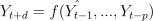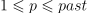# module timeseries.ar#

## Short summary#

module mlinsights.timeseries.ar

Auto-regressor for timeseries.

source on GitHub

## Classes#

class

truncated documentation

ARTimeSeriesRegressor

Base class to build a regressor on timeseries. The class computes one or several predictions at each time, between …

## Properties#

property

truncated documentation

_repr_html_

HTML representation of estimator. This is redundant with the logic of _repr_mimebundle_. The latter should …

## Methods#

method

truncated documentation

__init__

fit

Trains the model.

predict

Returns the prediction

## Documentation#

Auto-regressor for timeseries.

source on GitHub

class mlinsights.timeseries.ar.ARTimeSeriesRegressor(estimator='dummy', past=1, delay1=1, delay2=2, use_all_past=False, preprocessing=None)#

Base class to build a regressor on timeseries. The class computes one or several predictions at each time, between delay1 and delay2. It computes:with d in [delay1, delay2[ and.

source on GitHub

Parameters:
• estimator – estimator to use for regression, sklearn.linear_model.LinearRegression implements a linear auto-regressor, 'dummy' use past value as predictions

• past – values to use to predict

• delay1 – the model computes the first prediction for time=t + delay1

• delay2 – the model computes the last prediction for time=t + delay2 excluded

• use_all_past – use all past features, not only the timeseries

• preprocessing – preprocessing to apply before predicting, only the timeseries itselves, it can be a difference, it must be of type BaseReciprocalTimeSeriesTransformer

source on GitHub

__init__(estimator='dummy', past=1, delay1=1, delay2=2, use_all_past=False, preprocessing=None)#
Parameters:
• estimator – estimator to use for regression, sklearn.linear_model.LinearRegression implements a linear auto-regressor, 'dummy' use past value as predictions

• past – values to use to predict

• delay1 – the model computes the first prediction for time=t + delay1

• delay2 – the model computes the last prediction for time=t + delay2 excluded

• use_all_past – use all past features, not only the timeseries

• preprocessing – preprocessing to apply before predicting, only the timeseries itselves, it can be a difference, it must be of type BaseReciprocalTimeSeriesTransformer

source on GitHub

fit(X, y, sample_weight=None)#

Trains the model.

Parameters:
• X – output of X may be empty (None)

• y – timeseries (one single vector), array [n_obs]

• sample_weight – weights None or array [n_obs]

Returns:

self

source on GitHub

predict(X, y)#

Returns the prediction

source on GitHub